# EARTH SCIENCE LAB Plate Tectonics - Plate Motions Classroom Model

A number of mini-plate diagrams are shown below. The boundaries are numbered. Plate A is stationary. Determine the type of plate boundary that develops along each boundary during plate motion. Then select "Grade" to see if the boundaries have been correctly identified.

 Plate Motion #1: Starting Position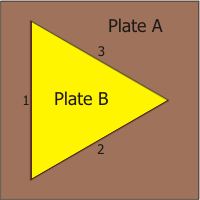Ending Position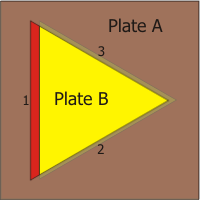Boundary #1 Convergent Divergent Transform Boundary #2 Convergent Divergent Transform Boundary #3 Convergent Divergent Transform

 Plate Motion #2: Starting PositionEnding Position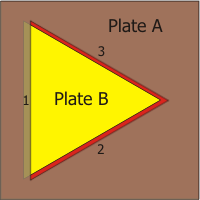Boundary #1 Convergent Divergent Transform Boundary #2 Convergent Divergent Transform Boundary #3 Convergent Divergent Transform

 Plate Motion #3: Starting PositionEnding Position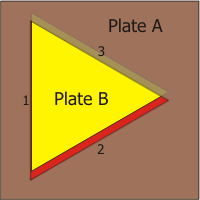Boundary #1 Convergent Divergent Transform Boundary #2 Convergent Divergent Transform Boundary #3 Convergent Divergent Transform

 Plate Motion #4: Starting PositionEnding Position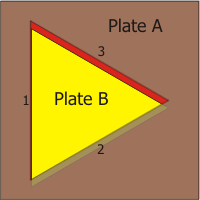Boundary #1 Convergent Divergent Transform Boundary #2 Convergent Divergent Transform Boundary #3 Convergent Divergent Transform

 Plate Motion #5: Starting PositionEnding PositionBoundary #1 Convergent Divergent Transform Boundary #2 Convergent Divergent Transform Boundary #3 Convergent Divergent Transform

 Plate Motion #6: Starting PositionEnding Position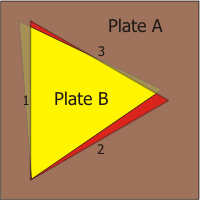Boundary #1 Convergent Divergent Transform Boundary #2 Convergent Divergent Transform Boundary #3 Convergent Divergent Transform

 Plate Motion #7: Starting PositionEnding Position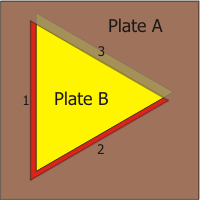Boundary #1 Convergent Divergent Transform Boundary #2 Convergent Divergent Transform Boundary #3 Convergent Divergent Transform

 Plate Motion #8: Starting PositionEnding Position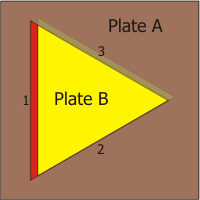Boundary #1 Convergent Divergent Transform Boundary #2 Convergent Divergent Transform Boundary #3 Convergent Divergent Transform

 Plate Motion #9: Starting PositionEnding Position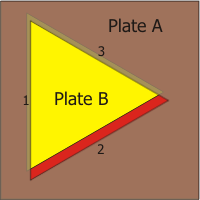Boundary #1 Convergent Divergent Transform Boundary #2 Convergent Divergent Transform Boundary #3 Convergent Divergent Transform

 Plate Motion #10: Starting PositionEnding Position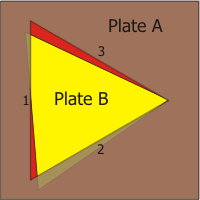Boundary #1 Convergent Divergent Transform Boundary #2 Convergent Divergent Transform Boundary #3 Convergent Divergent Transform

 Plate Motion #11: Starting Position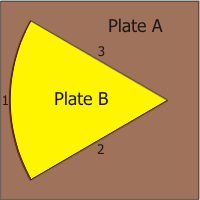Ending Position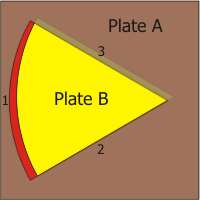Boundary #1 Convergent Divergent Transform Boundary #2 Convergent Divergent Transform Boundary #3 Convergent Divergent Transform

 Plate Motion #12: Starting PositionEnding PositionBoundary #1 Convergent Divergent Transform Boundary #2 Convergent Divergent Transform Boundary #3 Convergent Divergent Transform

 Plate Motion #13: Starting PositionEnding Position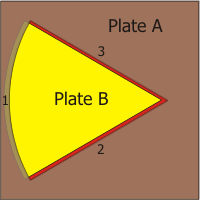Boundary #1 Convergent Divergent Transform Boundary #2 Convergent Divergent Transform Boundary #3 Convergent Divergent Transform

 Plate Motion #14: Starting PositionEnding Position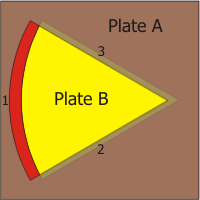Boundary #1 Convergent Divergent Transform Boundary #2 Convergent Divergent Transform Boundary #3 Convergent Divergent Transform

 Plate Motion #15: Starting PositionEnding Position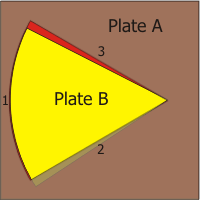Boundary #1 Convergent Divergent Transform Boundary #2 Convergent Divergent Transform Boundary #3 Convergent Divergent Transform

 Plate Motion #16: Starting PositionEnding Position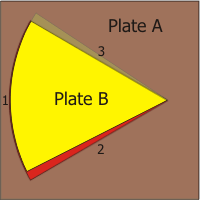Boundary #1 Convergent Divergent Transform Boundary #2 Convergent Divergent Transform Boundary #3 Convergent Divergent Transform